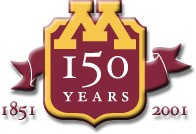# CSCI 5106: Programming Languages Using Pascal (Quick Guide)

To use the Pascal on our local cluster, you have to load the module soft/fpc. If you are new to the UMN setup and don't know about modules and how to load them, you can find some information about this in the system help pages; with specific regard to modules, you may look here.

Once you have loaded the module mentioned above, you can invoke the Pascal compiler by typing fpc.

To illustrate the use of this compiler, let us consider the following Pascal program that computes the sum of integers from 1 to 10:

```program sum;

{This program computes the  sum of integers from 1 to 10.}

const n	=  10;
var i, ssum : integer;

begin
ssum := 0;
i := n;
while (i > 0) do
begin
ssum := ssum + i;
i := i - 1;
end;
writeln(' The sum of integers from 1 to 10 is: ', ssum:5);
end.
```
Assume that this program is saved in a file with name sum.p (sum.pas would also work). You can then compile this program using the following command:
```% fpc sum.p
```
(The % symbol is the system prompt.) The compiler will then process the file sum.p, providing you with information as it goes along, some of which might be diagnostic in nature. If compilation is successful, as it should be in this case, an executable version will have been created in the file sum. You can run this as follows:
```% ./sum
The sum of integers from 1 to 10 is:   55
%
```
Sometimes you may want to produce an executable in a file with a different name from sum. You can force this by using the option -o with fpc. For instance, the command
```% fpc -osum.out sum.p
```
will put the executable in the file sum.out instead of in sum. There are some restrictions on the suffix or extension that can be used in file names that I do not understand completely. Look at the man page to find out about these, The compiler also has other switches that you can find out about from the man page.

Created by gopalan atsign cs dot umn dot edu. Maintained by ngopalan atsign umn dot edu and evw atsign umn dot edu. Last modified: August 30, 2019.

The views and opinions expressed in this page are strictly those of the page author(s). The contents of this page have not been reviewed or approved by the University of Minnesota.